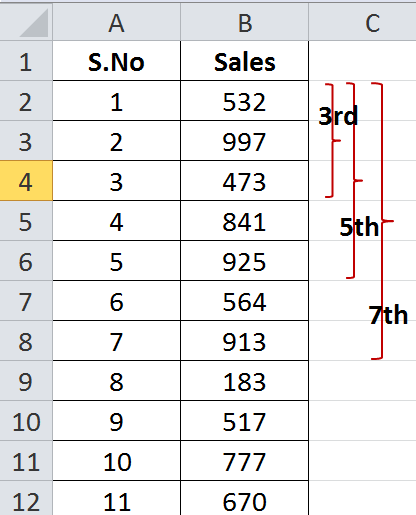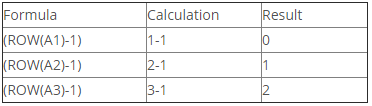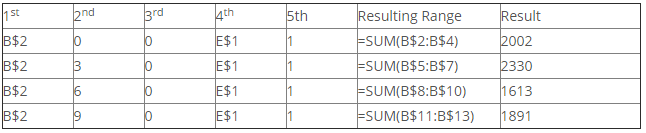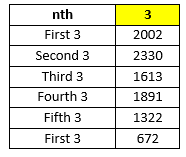# How To Sum Every Nth Row In ExcelIt is not uncommon that we want to sum data on the basis of certain criteria – on the basis of weeks, months, every tenth day and so on.  When we need to do it for larger data sets, we usually define start and the end points, but  when we are not sure about the start and then? points, we have to do it repeatedly. Repeatedly in the sense that we have to describe starting and the ending point for every period – week, month or any other period.So let’s consider a case where want to find the sum of (or consolidate sales for) every 3rd, 5th and 7th day of a sales at a super store. The data looks like following figure:

### The formula Used:

We will be using a combination of OFFSET() & SUM() to find the appropriate range of the data and then will sum it. For OFFSET(), we will be utilizing all the five arguments in its formula, and the SUM() will be working with Ctrl+Shift+Enter.

### The formula:

=SUM(OFFSET(B\$2,\$E\$1*(ROW(A1)-1),0,\$E\$1,1))

Let’s see how this formula works!

The formula starts by evaluating the inner most argument first i.e. ROW(A1)-1. As we drag down the formula, the formula results in the following array of values.This array of values (0, 1, 2,….n) is multiplied with the number-of-rows-to-be-summed i.e. 3 for this example.

This in return, creates another array of values – 0, 3, 6,…, 3n – all are multiple of 3.This series of value sets the second argument of the OFFSET() formula, since everything else in the formula remains the same, either due to fixed referencing or due to n-factor we can simplify the calculation as shown in the following table:

### OFFSET() function arguments and their workingWe can see from the above table that some arguments keep changing as we drag down the value and other are being kept constant. That generates a new range to sum every time and we have our desired result like one in the below table:This table concludes this post – that is all for now, will come with a new post next time. Thanks.